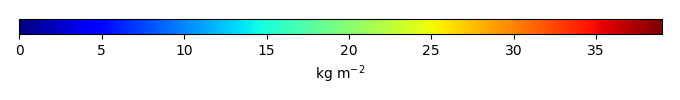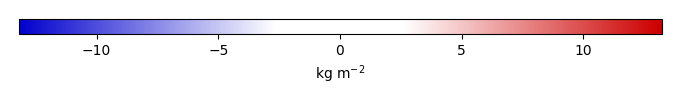# Mean State

Period Mean (original grids) [Pg]
Model Period Mean (intersection) [Pg]
Model Period Mean (complement) [Pg]
Benchmark Period Mean (intersection) [Pg]
Benchmark Period Mean (complement) [Pg]
Bias [kg m-2]
Bias Score 
Spatial Distribution Score 
Overall Score 
Benchmark [-] 26.2
CLM4 [-] 582. 21.9 560. 26.1 0.0878 -0.299 0.63 0.85 0.74
CLM4.5 [-] 560. 24.1 536. 26.1 0.0878 0.0184 0.62 0.87 0.74
CLM5 [-] 589. 21.7 567. 26.1 0.0878 -0.243 0.62 0.87 0.74
Period Mean (original grids) [Pg]
Model Period Mean (intersection) [Pg]
Model Period Mean (complement) [Pg]
Benchmark Period Mean (intersection) [Pg]
Benchmark Period Mean (complement) [Pg]
Bias [kg m-2]
Bias Score 
Spatial Distribution Score 
Overall Score 
Benchmark [-] 0.00338
CLM4 [-] 5.39 0.00986 5.08 0.00338 0.00 0.0933 0.62 0.68 0.65
CLM4.5 [-] 11.4 0.0180 11.1 0.00338 0.00 0.232 0.36 0.30 0.33
CLM5 [-] 11.9 0.0255 11.6 0.00338 0.00 0.302 0.36 0.40 0.38
Period Mean (original grids) [Pg]
Model Period Mean (intersection) [Pg]
Model Period Mean (complement) [Pg]
Benchmark Period Mean (intersection) [Pg]
Benchmark Period Mean (complement) [Pg]
Bias [kg m-2]
Bias Score 
Spatial Distribution Score 
Overall Score 
Benchmark [-] 17.5
CLM4 [-] 16.4 16.0 0.266 17.4 0.0333 -0.0421 0.67 0.91 0.79
CLM4.5 [-] 20.4 19.9 0.326 17.4 0.0333 0.973 0.68 0.90 0.79
CLM5 [-] 17.8 17.4 0.303 17.4 0.0333 0.386 0.67 0.91 0.79
Period Mean (original grids) [Pg]
Model Period Mean (intersection) [Pg]
Model Period Mean (complement) [Pg]
Benchmark Period Mean (intersection) [Pg]
Benchmark Period Mean (complement) [Pg]
Bias [kg m-2]
Bias Score 
Spatial Distribution Score 
Overall Score 
Benchmark [-] 0.222
CLM4 [-] 25.1 0.735 24.5 0.222 0.00 6.06 0.35 0.40 0.37
CLM4.5 [-] 34.8 0.804 34.2 0.222 0.00 6.81 0.33 0.46 0.40
CLM5 [-] 56.8 0.907 56.1 0.222 0.00 8.04 0.29 0.39 0.34
Period Mean (original grids) [Pg]
Model Period Mean (intersection) [Pg]
Model Period Mean (complement) [Pg]
Benchmark Period Mean (intersection) [Pg]
Benchmark Period Mean (complement) [Pg]
Bias [kg m-2]
Bias Score 
Spatial Distribution Score 
Overall Score 
Benchmark [-] 8.47
CLM4 [-] 5.11 5.12 0.0325 8.42 0.0520 -0.736 0.57 0.57 0.57
CLM4.5 [-] 3.42 3.42 0.0387 8.42 0.0520 -1.16 0.49 0.37 0.43
CLM5 [-] 3.49 3.46 0.0505 8.42 0.0520 -1.12 0.51 0.70 0.60

# Temporally integrated period mean

BENCHMARK MEANMODEL MEANBIASBIAS SCORESPATIAL TAYLOR DIAGRAMMODEL COLORS# Spatially integrated regional mean

MODEL COLORS# All Models

BenchmarkCLM4CLM4.5CLM5